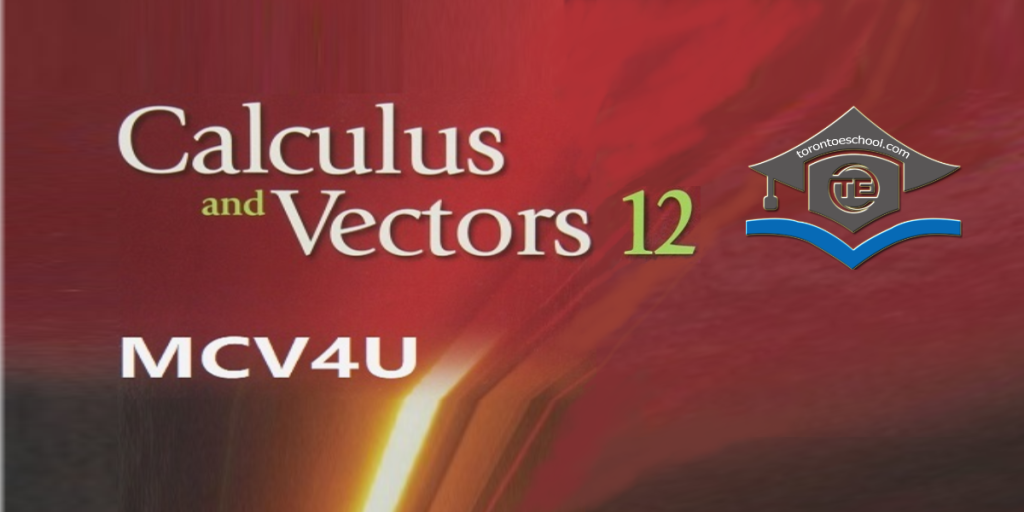# MCV4U Calculus & Vectors Grade 12 Online Course## MCV4U Calculus and Vectors Grade 12

MCV4U Calculus & Vectors Grade 12 is one of the major compulsory courses for most majors of admission to all universities. Get ready for university applications in Ontario with Toronto eSchool’s Grade 12 Calculus and Vectors course.

Course Title: Calculus and Vectors, Grade 12, University Preparation
Course Name: Calculus and Vectors
Course Code: MCV4U
Course Type: University Preparation
Credit Value: 1.0
Prerequisite: Advanced Functions MHF4U ( may be taken concurrently )
Curriculum Policy Document: Mathematics, The Ontario Curriculum, Grades 11 and 12, 2007 (Revised)
Course Developer: Toronto eSchool
Department: Mathematics
Department Head: Sofika Haxhi, M.Sc., B.Ed., OCT
Developed Date: 2014
Most Recent Revised Date: 2022

Register Now

Course Description:

MCV4U – Calculus and Vectors course builds on students’ previous experience with functions and their developing understanding of rates of change. Students will solve problems involving geometric and algebraic representations of vectors and representations of lines and planes in three-dimensional space; broaden their understanding of rates of change to include the derivatives of polynomial, sinusoidal, exponential, rational, and radical functions; and apply these concepts and skills to the modelling of real world relationships. Students will also refine their use of the mathematical processes necessary for success in senior mathematics. This course is intended for students who choose to pursue careers in fields such as science, engineering, economics, and some areas of business, including those students who will be required to take a university-level calculus, linear algebra, or physics course.

Unit Unit Titles and Descriptions Time and Sequence
1 Introduction to Calculus
A variety of mathematical operations with functions are needed in order to do the 12 hours calculus of this course. This unit begins with students developing a better understanding of these essential concepts. Students will then deal with rates of change problems and the limit concept. While the concept of a limit involves getting close to a value but never getting to the value, often the limit of a function can be determined by substituting the value of interest for the variable in the function. Students will work with several examples of this concept. These basic ideas will be extended and expanded to be able to distinguish between average and instantaneous rates of change to help students solve problems arising in real-world applications.
12 hours
2 Derivatives
The concept of a derivative is, in essence, a way of creating a short cut to determine the tangent line slope function that would normally require the concept of a limit. Once patterns are seen from the evaluation of limits, rules can be established to simplify what must be done to determine this slope function. This unit begins by examining those rules including: the power rule, the product rule, the quotient rule and the chain rule followed by a study of the derivatives of composite functions.
14 hours
3 Derivatives of Exponential, Logarithmic and Trigonometric Functions
In this unit, students will learn about the rates of change of exponents and Logarithms. Students will cover derivatives of exponential functions and its applications. Students will study derivatives of Logarithmic and Trigonometric Functions as well.
13 hours
4 Curve Sketching
Determine maximum and minimum values of the graphs of polynomial functions using first derivative test; use the second derivative test to determine the intervals of concavity; sketch the graph of a polynomial function, given its equation, by using 5 steps. This unit will help you understand the fundamentals of using calculus to help us sketch curves, and solve some basic optimization problems.
13 hours
5 Derivative Applications and Related Rates
Students will learn about how to calculate Implicit and Logarithmic differentiation; apply the concepts of derivative to sketch the velocity-time and acceleration-time graph; solve problems arising from real world applications, such as population and rates of population change, volume and rates of flow, height and growth rates.
9 hours
6 Introduction to Vectors
In this unit, students will learn to define a vector as a quantity with both magnitude and direction; distinguish between a scalar quantity and vector quantity; add, subtract vectors both graphically and algebraically; represent a vector in two-space using angle system, directional system, and bearing system and solve real world problems involving operations with vectors in two dimensions.
9 hours
7 Vector Applications
Applications involving work and torque are used to introduce and lend context to the dot and cross products of Cartesian vectors. The vector and scalar projections of Cartesian vectors are written in terms of the dot product. The properties of vector products are investigated and proven. These vector products will be revisited to predict characteristics of the solutions of systems of lines and planes in the intersections of lines and planes.
12 hours
8 Lines in Three-Space
A variety of types of problems exist in this unit and are generally grouped into the following categories: Pythagorean Theorem Problems (these include ladder and intersection problems), Volume Problems (these usually involve a 3-D shape being filled or emptied), Trough Problems, Shadow problems and General Rate Problems. During this unit students will look at each of these types of problems individually.
9 hours
9 Planes
In this unit, students will recognize a normal to a plane geometrically and algebraically; identify the cases when two planes coincide or they are two distinct parallel planes and determine the equation of a plane that intersect with two other planes of planes in one point, or in more than one point.
9 hours
10 Matrices and Linear Systems
This unit teach students to convert linear systems to matrix; add, subtract and multiply matrices and determine the points of intersections of three planes using operations with matrices. The unit ends, as in all other units, with an quiz and a unit test.
8 hours
Final Assessment
Final Exam